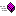XLL+ Class Library (7.0)

# Global conversion functions

Global conversion functions are listed here.

#### Remarks

For a detailed discussion of the conversion functions see the technical note Conversion functions.

#### ConversionXlReadGroupedScalar Transform one item in a grouped CXlOper into a single valueXlReadGroupedScalarEx Transform one item in a grouped CXlOper into a single value of an extended typeXlReadGroupedVector Transform one row or column of a CXlOper into a vectorXlReadGroupedVectorEx Transform one row or column of a CXlOper into a vector of an extended typeXlReadMatrix Transform a CXlOper into a matrixXlReadMatrixEx Transform a CXlOper into a matrix of an extended typeXlReadScalar Transform a CXlOper into a single valueXlReadScalarEx Transform a CXlOper into a single value of an extended typeXlReadVector Transform all or part of a CXlOper into a vectorXlReadVectorEx Transform all or part of a CXlOper into a vector of an extended type

#### Conversion of return valuesXlWriteMatrixEx Transform a matrix of extended type values into a CXlOperXlWriteScalarEx Transform a single value of extended type into a CXlOperXlWriteVectorEx Transform a vector of extended type values into a CXlOper